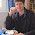## Sunday, June 6, 2010

### Lost theorem about angular proportions

Last week, I came across a so called missing theorem about angular proportions in a triangle, discovered (or rediscovered) by Leon Romain. This triangle construction is presented by user Linelites on youtube.

For such a "Romain triangle", the missing theorem states that, if one of the inner angles is twice another inner angle, one has the property a² = bc +c², where a, b and c are outlined in Figure 1.

The sine chord pattern presented in the Teaching sine function with spaghetti provides helpful insights for the missing theorem. In this pattern, all angles at the intersection of chords are integer multiples of a chosen unit angle or its complement, modulo 90°. All segment lengths in this pattern can therefore be written as sums or differences of cosine and sine products and ratios of that angle. Figure 2 pictures some measures of sine chords in a circle of unit diameter, for an arbitrary angle θ.

Figure 3 shows a Romain triangle for angle α in this pattern. There are numerous other Romain triangles in this pattern. Can you figure them out? With the help of the measures pictured in Figure 2, one can follow visually the elements of a proof for the missing theorem.

Side b is the sine of the chosen angle θ (Figure 4):

b = sinθ

Side c times sin equals b times sinθ (Figure 5):

c = sin²θ/sin

Side a equals b cosθ minus c cos (Figure 6)

a = sinθ cosθ - sin²θ cos /sin

Working out a² and bc +c², one finds that they are both equal to 2 sin²θ cosθ / sin3θ.

The interesting thing is that the sine chord pattern hosts plenty of "missing theorems" about angular proportions, which are only waiting to be (re)discovered.

______________________
Update (June 7, 2010): I corrected Figure 6, which held a wrong term.

#### 3 comments:

1.Dear Arjen,

It's been a somewhat winding trek, starting from a general googling on quantum physics, on to the "Making sense" page on Wikiversity, and then, being impressed, trying to get to know the author of that Wiki page by visiting its history page, and then, after a couple of further links, landing in here. Gotcha!!

Am still downloading materials off your Materion site, but already think that I could have an informal exchange via emails with you.

If interested, drop me an email at: aj175tp [at] yahoo [dot] co [dot] in.

Regards,

--Ajit
http://www.JadhavResearch.info
http://AjitJadhav.wordpress.com
http://www.iMechanica.org/user/1150

2.Label the triangle ABC, so that 'A' is the angle opposite side 'a', 'B' is the angle opposite side 'b', etc. Let circle ABC be circumscribe about the triangle ABC; so the three sides of the triangle become chords cutting off arcs AB, arc BC, and arc ABC. Notice that arc AB = theta, arc BC = twice theta, and arc ABC = thrice theta. Bisect arc BC at D and draw the lines AD, BD, and CD. Since arc AB = arc BD = arc DC, therefore, chord AB = chord BD = chord DC = 'c'. Draw triangle ACD. It is congruent with triangle ABC. Draw inscribed quadrilateral ABDC. Its diagonals both equal 'a', three sides equal 'c' and one side equals 'b'.
Now, by Ptolemy's Theorem of the Inscribed Quadrilateral, the products of the diagonals equals the sum of the products of the opposite sides. Therefore, a^2 = c^2 + bc. Q.E.D.

3.Hi Ajit,
Just leaving a comment. We've been sharing ideas by mail.

Hi Peter,
That's an elegant proof. Thanks!